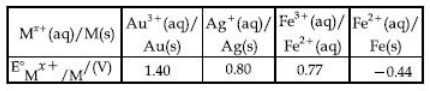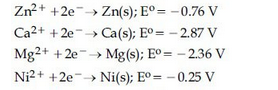## Filters

Sort by :
Clear All
Q

An example of a disproportionation reaction is :

• Option 1)

• Option 2)

• Option 3)

• Option 4)

Disproportionation Reactions - A special type of redox reaction in which one oxidation state is simultaneously oxidised and reduced. One of the reacting substances in a disproportionation reaction always contains an element that can exist in at least three oxidation states.  - wherein +1     -1                     +1    -2               0       Disproportionation reactions are those where...

Consider the statements S1 and S2 :

S1 : Conductivity always increases with decrease in the concentration of electrolyte.

S2 : Molar conductivity always increases with decrease in the concentration of electrolyte.

The correct option among the following is :

• Option 1)

Both S1 and S2 are wrong

• Option 2)

S1 is wrong and S2 is correct

• Option 3)

Both S1 and S2 are correct

• Option 4)

S1 is correct and S2 is wrong

Molar conductivity is given as   , K = conductivity & c = concentration We know that  Conductivity increases with increase in concentration.  Molar Conductivity increases with decrease in concentration.  S1 is wrong & S2 is correct.  option (2) is correct.Option 1)Both S1 and S2 are wrongOption 2)S1 is wrong and S2 is correctOption 3)Both S1 and S2 are correctOption 4)S1 is correct and S2 is wrong

ven :

Oxidising power of the species will increase in the  order:

• Option 1)

• Option 2)

• Option 3)

• Option 4)

Electrode Potential - The potential associated with each electrode is known as electrode potential. -     Electro-chemical series - The standard reduction potential of a large number of electrodes have been measured using standard hydrogen electrode as the reference electrode. These various electrode can be arranged in increasing electrode potential. -       Standard Electrode Potential Value...

Which one of the following graphs between molar conductivity  versus  is correct ?

• Option 1)• Option 2)• Option 3)• Option 4)electrolyte have higher value of   w..r.t   because  ionise more hydrated w.r.t  ion Option 1)Option 2)Option 3)  Option 4)

A solution of  is electrolysed between platinium electrodes using 0.1 faraday electricity . How many mole of  will be deposited at the cathode ?

• Option 1)

• Option 2)

• Option 3)

• Option 4)

Faraday's first law of electrolysis - The mass of any substance deposited or dissolved at any electrode during electrolysis is directly propotional to the quantity of electricity passed through the solution. - wherein W = Z I t Z = mass of electrolyte get deposited Z = electro chemical equivalent                need 2 mole of   for 1 mole of   Option 1) Option 2) Option 3) Option 4)

The standard Gibbs energy for the given cell reaction in  at  is :

• Option 1)

• Option 2)

• Option 3)

• Option 4)

We know,                                                         Option 1)   Option 2)   Option 3) Option 4)

The correct order of the oxidation states of nitrogen in  and  is :

• Option 1)

• Option 2)

• Option 3)

• Option 4)

Option 4

Calculate the standard cell potential (in V) of the cell in which following reaction takes place:

Given that

• Option 1)

x-z

• Option 2)

x-y

• Option 3)

x+2y-3z

• Option 4)

x+y-z

Given              Cell equation :-                             (Oxidation)                    (Cathode Reduction)  Std. EMF of given cell reaction:-              (iii-ii)     Put this in eqn (i)  Option 1)x-zOption 2)x-yOption 3)x+2y-3zOption 4)x+y-z

Given that

The strongest oxidizing agent is :

• Option 1)

• Option 2)

• Option 3)

• Option 4)

For strongest oxidising agent, standard reduction potential should be highest and so, it is highest for .  Option 1)Option 2)Option 3)  Option 4)
Potassium permanganate is an inorganic chemical compound with the formula KMnO4. It is a salt consisting of K+ and MnO4 − ions.It known as permanganate of potash, it is a strong oxidizing agent. It dissolves in water to give intensely purple solutions.
Screenshot_1152.pngE Online Coaching for IIT JEE Mair X E Online Coaching for IIT JEE Mair X Take free online mock test, Get? X View oven.'iew analysis report cf C a https://www.onlinetestseries.in/allenkota/test-center/overview?id=5071&type=mock&pckg_id=456&eia=1 Right Answer: 4 | Your Answer: 4 Difficulty Level: medium I Topic: chemistry > > halogen derivative Peer Stat(%): Unattempted-26.4 Correct-37.g Incorrect-35.7 In which of the following reaction Saytzeff alkene is major product? Right Answer: 4 | Your Answer: 3 Difficulty Level: medium I Topic: chemistry halogen derivative Peer Stat(%): Unattempted-46.6 Correct-24.1 Incorrect-29.3 Anisole Product : x o 4/4 -1/4 View In : 1125 1135 View View Q No: 43 Standard EC of the half cell Fe Fez * (1) Cu oxidises Fez* ion (2) Cu2* oxidises Fe (3) Cu reduces Fez* ion Complete Question Content is +0.44 V and standard EC of half cell Cu Cu2* is -0.32 V. Then : Close English (4) Cu2* reduces Fe Correct Answer: 2 Status: unattempted 45. 21:32 20-02-2019 46. Add to Bookmark List Difficulty Level: medium I Topic: chemistry > > electrochemistry Peer Stat(%): Unattempted-18.4 Correct-64.2 Incorrect-17.4 Equivalent conductivity of BaC12, H2S04 and HCI, are xl, x2 and x3 S cm2 Right Answer: 3 | Your Answer: Difficulty Level: high I Topic: chemistry electrochemistry Peer Stat(%): Unattempted-63.5 Correct-g.4 Incorrect-27.1 using same quantity of current, which among Na, Mg and A1 Right Answer: 3 | Your Answer: I Difficulty Level: medium I Topic: chemistry > > electrochemistry Peer Stat(%): Unattempted-20.8 Correct-47.8 Incorrect-31.4 is deposited -1/4 Isos Isss cti 1295 View View a'e W ndows ot Settncs o activ?te Offline - Offline, available between 10 .
different electrons we use dg =-nfe
Screenshot_1175.pngE Online Coaching for JEET X E Online Coaching for JEET X 15507906477791354857386 C a https://www.onlinetestseries.in/allenkota/test-center/overview?id=5071&type= X Take free online mock test: an s an View overview analysis repc x Q O an ar e a ce e Right Answer: 2 | Your Answer: Difficulty Level: low I Topic: chemistry > > electrochemistry Peer Stat(%): Unattempted-27.3 Correct-40.4 Incorrect-32.3 In Q. If Q Keq then which one is not correc Nernst equation is E = EO Right Answer: 3 | Your Answer: Difficulty Level: medium I Topic: chemistry > > electrochemistry Complete Question Content 43 gs View o 0/4 180s View Close English Q No: 47 Calulate EO Mn02/Mnoa given EO Mn /Mnoa (1) 1.69 v (2) 1.69 (3) 0.28 (4) -0.28 Correct Answer: Your Answer:4 Status: incorrect -1.51 V and EO Mn02/Mn 1.23 v View In : Add to Bookmark List Peer Stat(%): Unattempted-37.3 Correct-45.6 Incorrect-17.2 The mass of glucose that should be dissolved in 100 g of water in order to 11S View View View View View 11:18 22-02-2019 Right Answer: 3 | Your Answer: Difficulty Level: low I Topic: chemistry > > liquid solution Peer Stat(%): Unattempted-35.5 Correct-58.2 Incorrect-6.3 Mole fraction of a non electrolyte in aqueous solution is 0.07. Right Answer: 3 | Your Answer: Difficulty Level: medium I Topic: chemistry > > liquid solution Peer Stat(%): Unattempted-4g.2 Correct-37.3 Incorrect-13.5 If Kf is 1 Go tc _ Which one of the following pair of solution are not isotonic at same tempe Right Answer: 3 | Your Answer: 2 Live Support May I Help You? Online - Click here to chat
New_Doc_2019-02-07_15.05.07_20.jpg

Screenshot_1152.pngThe Standard E0 value of half cell Fe Fe2 is:

Consider the following standard electrode potentials (E0 in volts) in aqueous solution :Based on these data, which of the following statements is correct ?

• Option 1)

Tl+ is more stable than Al3+

• Option 2)

Al+ is more stable than Al3+

• Option 3)

Tl+ is more stable than Al+

• Option 4)

Tl3+ is more stable than Al3+

Option 1) Tl+ is more stable than Al3+ Option 2) Al+ is more stable than Al3+ Option 3)  Tl+ is more stable than Al+ Option 4) Tl3+ is more stable than Al3+
Screenshot_953.pngE Formula of Molar Conductivityâ€” X E Online Coaching for IIT JEE Mair X E Online Coaching for IIT JEE Mair X E Online Coaching for IIT JEE Mair X + C https://www.entrance360.com/redox-reaction-and-electrochemistry/formula-of-molar-conductivity_4875/cc/?concept_ids=&exam=jee-main#questions Watch our TV Ad Engineering Medical Govemment Jobs â€¢ Q&A Dashboard santhosh Logout Ask a Question Ask or Search for exams, articles, questions Formula of Molar Conductivity - (Concept) cmÂ¯l Am(S cm2molÂ¯l) = x molarity (mol Videos Question Exam Questions Books Resistance of a cell of 0.1M solution is 100 ohm. While its conductivity is 1.29 Sm-l conductivity of 0.02 M solution of the electrolyte will be Resistance of the same cell filled with 0.2M of the same solution is 520 ohm. The molar c. O O O O 1.24 x 10 124 x lo- 1240 x 10 12.4 x 10 Sm2molÂ¯l 4 Sm2molÂ¯l Sm2molÂ¯l Sm2molÂ¯l https:,:,%'.â€¢'e.â€¢â€¢.v.sntrencÂ±?f.ccâ€¢m,'lÂ±dcx-reacticâ€¢n-znd-Â±lsct-c&,emiftrâ€¢/,:fcâ€¢rmua-af-mcâ€¢'Ã¤'-ccnclue'.â€¢it-v' ' ; 7, -ncecâ€¢t Activate Windows Next Â» ENG US 13:00 05-02-2019

For the cell , different half cells and their standerd electrode potential are given below :If which cathode will give a maximun value of   per electron transfered?

• Option 1)

• Option 2)

• Option 3)

• Option 4)

Standard Electrode Potential Value - As we know that E0cell is maximum with E0Au+3/Au(s) among others In thecell reaction elecrons transfered quantity doesn't matter. -    Option 1)Option 2)Option 3)Option 4)

Given :

Based on the above thermochemical equations, find out which one of the following algebraic relationships is correct ?

• Option 1)

• Option 2)

• Option 3)

• Option 4)

Electrochemistry - It is the study of production of electricity from energy released during spontaneous chemical reactions and the use of electrical energy to bring about non spontaneous chemical transformations. Balancing of Redox Reactions - Oxidation number method - wherein 1. Write the correct formula for each reactant and product. 2. Identify atom whose oxidation number is changed. 3....

In the reaction of oxalate with permannganate in acidic medium, the number of electrons involved in producing one molecule of is:

• Option 1)

10

• Option 2)

2

• Option 3)

1

• Option 4)

5

Balancing of Redox Reactions - Oxidation number method - wherein 1. Write the correct formula for each reactant and product. 2. Identify atom whose oxidation number is changed. 3. Calculate increase / decrease in oxidation number per atom and for the entire molecule / ion in which it occures. 4. Add  on appropriate side so that the total ionic charges of reactants and products are equal. 5....

In the cell

the cell potential is 0.92V when a 10-6 molal solution is used . The stansard electrode potential of electrode is:

• Option 1)

0.20 V

• Option 2)

0.40V

• Option 3)

0.76V

• Option 4)

0.94V

Nernst Equation - - wherein is concentration of species F= 96487 C  R= 8.314  T= Temperature in kelvin   As we learned in cell potential At Anode : At Cathode : = 0.2 V  Option 1)0.20 VOption 2)0.40VOption 3)0.76VOption 4)0.94V

Consider the following reduction processesThe reducing power of the metals increases in order :

• Option 1)

Ca < Zn < Mg < Ni

• Option 2)

Ni < Zn < Mg < Ca

• Option 3)

Zn < Mg < Ni < Ca

• Option 4)

Ca < Mg < Zn < Ni

Reduction - Removal of Oxygen / electronegative element from a substance of addition of hydrogen / electropositive element to substance. - wherein e.g.       Electrode Potential - The potential associated with each electrode is known as electrode potential. -     Standard Electrode Potential Value - -     Standard reduction potential - It measure the tendency of a chemical species to acquire...
Exams
Articles
Questions